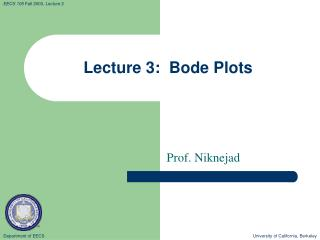Download PresentationLecture 3: Bode Plots

Loading in 2 Seconds...

# Lecture 3: Bode Plots - PowerPoint PPT Presentation

Lecture 3: Bode Plots. Prof. Niknejad. Get to know your logs!. Engineers are very conservative. A “margin” of 3dB is a factor of 2 (power)!I am the owner, or an agent authorized to act on behalf of the owner, of the copyrighted work described.
Download Presentation## Lecture 3: Bode Plots

An Image/Link below is provided (as is) to download presentation

Download Policy: Content on the Website is provided to you AS IS for your information and personal use and may not be sold / licensed / shared on other websites without getting consent from its author.While downloading, if for some reason you are not able to download a presentation, the publisher may have deleted the file from their server.

- - - - - - - - - - - - - - - - - - - - - - - - - - E N D - - - - - - - - - - - - - - - - - - - - - - - - - -
Presentation Transcript
1. Lecture 3: Bode Plots Prof. Niknejad

2. Get to know your logs! • Engineers are very conservative. A “margin” of 3dB is a factor of 2 (power)! • Knowing a few logs by memory can help you calculate logs of different ratios by employing properties of log. For instance, knowing that the ratio of 2 is 3 dB, what’s the ratio of 4? University of California, Berkeley

3. Bode Plot Overview • Technique for estimating a complicated transfer function (several poles and zeros) quickly • Break frequencies : University of California, Berkeley

4. Summary of Individual Factors • Simple Pole: • Simple Zero: • DC Zero: • DC Pole: University of California, Berkeley

5. Example • Consider the following transfer function • Break frequencies: invert time constants University of California, Berkeley

6. Breaking Down the Magnitude • Recall log of products is sum of logs • Let’s plot each factor separately and add them graphically University of California, Berkeley

7. Breaking Down the Phase • Since • Let’s plot each factor separately and add them graphically University of California, Berkeley

8. 80 60 40 0 dB 20 104 105 106 107 108 109 1010 1011 -20 -40 -60 -80 Magnitude Bode Plot: DC Zero University of California, Berkeley

9. 180 135 90 45 104 105 106 107 108 109 1010 1011 -45 -90 -135 -180 Phase Bode Plot: DC Zero University of California, Berkeley

10. 80 60 40 20 104 105 106 107 108 109 1010 1011 -20 -40 -60 -80 Magnitude Bode Plot: Add First Pole University of California, Berkeley

11. 180 135 90 45 104 105 106 107 108 109 1010 1011 -45 -90 -135 -180 Phase Bode Plot: Add First Pole University of California, Berkeley

12. 80 60 40 20 104 105 106 107 108 109 1010 1011 -20 -40 -60 -80 Magnitude Bode Plot: Add 2nd Zero University of California, Berkeley

13. 180 135 90 45 104 105 106 107 108 109 1010 1011 -45 -90 -135 -180 Phase Bode Plot: Add 2nd Zero University of California, Berkeley

14. 80 60 40 20 104 105 106 107 108 109 1010 1011 -20 -40 -60 -80 Magnitude Bode Plot: Add 2nd Pole University of California, Berkeley

15. 180 135 90 45 104 105 106 107 108 109 1010 1011 -45 -90 -135 -180 Phase Bode Plot: Add 2nd Pole University of California, Berkeley

16. Comparison to “Actual” Mag Plot University of California, Berkeley

17. Comparison to “Actual” Phase Plot University of California, Berkeley

18. Why do I say “actual”? • I plotted the transfer characteristics with Mathematica • The range of frequency for the plot is 6 orders of magnitude. The program has to find the “hot spots” in order to plot the function. Near the hot spots, more points are plotted. In between hot spots, the function is interpolated. If you pick the wrong points, you’ll end up with the wrong plot: • mag = LogLinearPlot[20*Log[10, Abs[H[x]]], {x, 10^4, 10^11},PlotPoints -> 10000, Frame -> True,PlotStyle -> Thickness[.005], ImageSize -> 600,GridLines -> Automatic, PlotRange -> {{10^4, 10^11}, {-20, 100}} ] University of California, Berkeley

19. Don’t always believe a computer! University of California, Berkeley

20. Second Order Transfer Function • The series resonant circuit is one of the most important elementary circuits: • The physics describes not only physical LCR circuits, but also approximates mechanical resonance (mass-spring, pendulum, molecular resonance, microwave cavities, transmission lines, buildings, bridges, …) University of California, Berkeley

21. Series LCR Analysis • With phasor analysis, this circuit is readily analyzed + Vo − University of California, Berkeley

22. + Vo − Second Order Transfer Function • So we have: • To find the poles/zeros, let’s put the H in canonical form: • One zero at DC frequency  can’t conduct DC due to capacitor University of California, Berkeley

23. Poles of 2nd Order Transfer Function • Denominator is a quadratic polynomial: University of California, Berkeley

24. Im Re Finding the poles… • Let’s factor the denominator: • Poles are complex conjugate frequencies • The Q parameter is called the “quality-factor” or Q-factor • This parameters is an important parameter: University of California, Berkeley

25. Im Re Resonance without Loss • The transfer function can parameterized in terms of loss. First, take the lossless case, R=0: • When the circuit is lossless, the poles are at real frequencies, so the transfer function blows up! • At this resonance frequency, the circuit has zero imaginary impedance • Even if we set the source equal to zero, the circuit can have a steady-state response University of California, Berkeley

26. Magnitude Response • The response peakiness depends on Q University of California, Berkeley